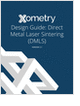MaterialsDesign CenterProcessesUnit ConversionFormulasMathematicsCalculatorsDiscussion ForumTrade PublicationsDirectory Service
Metal 3D Printing Design Guide

Direct Metal Laser Sintering (DMLS) 3D printing for parts with reduced cost and little waste.

Learn the best principles to negotiate the salary you deserve!

3D Scanners

A white paper to assist in the evaluation of 3D scanning hardware solutions.

Salary Expectation

8 things to know about the interview question "What's your salary expectation"?

more free publicationsGlossary » Units » Energy » QuadrillionQuadrillion (quad) is a unit in the category of Energy. It is also known as quadrillions. This unit is commonly used in the US unit system. Quadrillion (quad) has a dimension of Ml2T-2 where M is mass, L is length, and T is time. It can be converted to the corresponding standard SI unit J by multiplying its value by a factor of 1.05505585262E+018.
Note that the seven base dimensions are M (Mass), L (Length), T (Time), Q (Temperature), N (Aamount of Substance), I (Electric Current), and J (Luminous Intensity).

Other units in the category of Energy include A.u. of Energy (Eh), Barrel Oil Equivalent (bboe), Bboe (barrel Oil Equivalent) (bboe), BeV (billion EV) (BeV), British Thermal Unit (ISO) (Btu (ISO)), British Thermal Unit (IT) (Btu (IT)), British Thermal Unit (mean) (Btu (mean)), British Thermal Unit (thermochemical) (Btu (therm.)), Calorie (15°C) (cal15), Calorie (4°C) (cal4), Calorie (diet Kilocalorie) (Cal, kcal), Calorie (IT) (International Steam Table) (cal (IT)), Calorie (mean) (calmean), Calorie (thermochemical) (cal (therm.)), Celsius-Heat Unit (Chu), Coulomb Volt (C-V), Cubic Centimeter-Atm (cm3-atm), Cubic Foot Atm (ft3-atm), Electronvolt (eV), Erg (erg), Foot-Pound Force (ft-lbf), Foot-Poundal (ft-pdl), Gigaelectronvolt (GeV), Gram Calorie (gram-cal), Hartree (Eh), Horsepower (550ft-Lbf/s)-Hour (Hp-h), Inch Pound Force (in-lbf), Joule (J), Kilocalorie (15°C) (kcal15), Kilocalorie (4°C) (kcal4), Kiloelectronvolt (keV), Kilojoule (kJ), Kiloton TNT Equivalent (kt (TNT)), Kilowatt-Hour (kWh), Megaelectronvolt (MeV), Megajoule (MJ), Megaton TNT Equivalent (Mt (TNT)), Newton Meter (N-m), Q Unit, Quad (quad), Rydberg (Ry), Tce (tonne Coal Equivalent) (tce), Therm (EEG), Therm (US), Thermie (15°C) (th15 °C), Toe (tonne Oil Equivalent) (toe), Ton TNT Equivalent (ton (TNT)), Tonne Coal Equivalent (tce), Tonne Oil Equivalent (toe), and Watt Hour (Wh).N/ARelated PagesGlossaryMetal 3D Printing Design Guide

Direct Metal Laser Sintering (DMLS) 3D printing for parts with reduced cost and little waste.